# 第二章 贝叶斯分类器

### 1 贝叶斯公式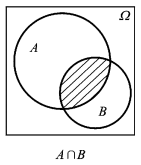P(AB)=P(B|A)P(A)=P(A|B)P(B)得:

P(B|A) = P(A|B)P(B)/P(A)

P(h|D）=P(D|h)P(h)/P(D)
P(h)表示在没有训练数据前假设h拥有的初始概率。P(h)被称为h的先验概率。先验概率反映了关于h是一正确假设的机会的背景知识。

### 2 如何理解贝叶斯公式

P(B|A)=P(A|B)P(B)/P(A)

### 3 全概率公式

BiBj=(ij)B1+B2+=Ω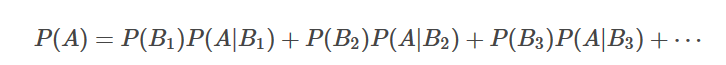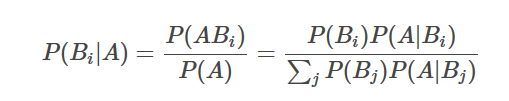### 4 贝叶斯决策论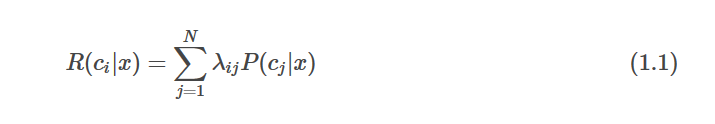### 什么是期望损失（条件风险）？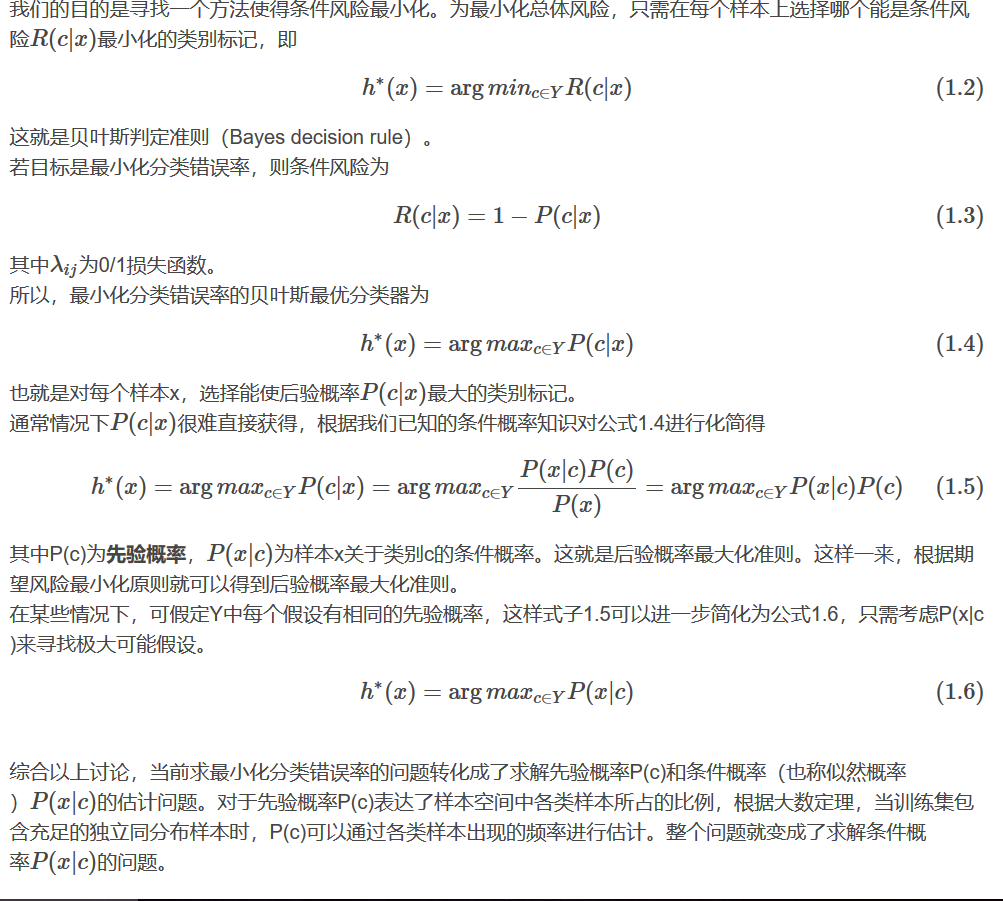### 5 极大似然估计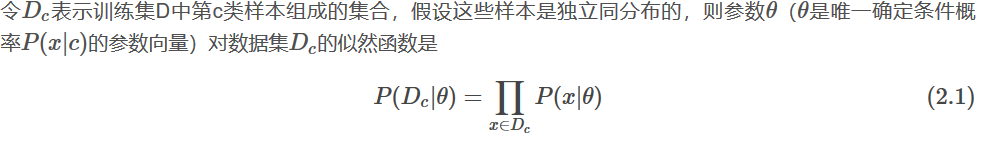### 6 朴素贝叶斯分类器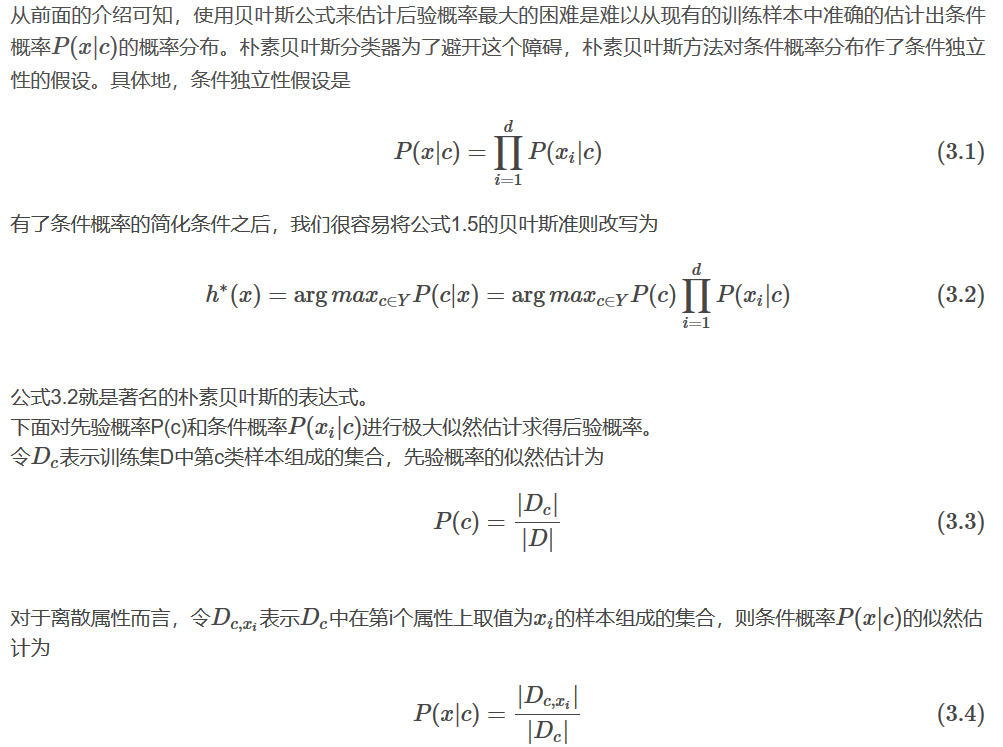### 10 贝叶斯网的学习©️2019 CSDN 皮肤主题: 大白 设计师: CSDN官方博客